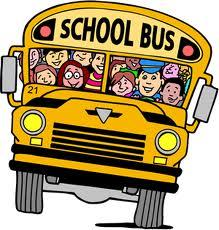# Interested 3512

One-third of the 8A class student wants to go to high school after finishing primary school, and half of the students are interested in the field of study. The remaining four have not yet been decided. How many students are there in 8A?

n =  24

### Step-by-step explanation:

n/3+n/2+4=n

n = 24

n = 24/1 = 24

n = 24

Our simple equation calculator calculates it.Did you find an error or inaccuracy? Feel free to write us. Thank you!

Tips for related online calculators
Do you have a linear equation or system of equations and looking for its solution? Or do you have a quadratic equation?